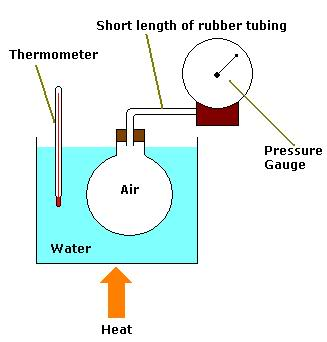# Pressure Law - question about experiment apparatus

• slakedlime

#### slakedlime

[SOLVED] Pressure Law - question about experiment apparatusIntroduction
Above is a diagram representing the apparatus which can be used to perform the experiment which verifies the Pressure Law (i.e. pressure is directly proportional to temperature for a fixed mass and volume of gas).

The question
Why is it important to use a short length of rubber tubing to connect the flask to the pressure gauge?

The attempt at a solution Here is my hypothesis:
The rubber tubing contains a volume of air which is not accounted for in the experiment (the volume of air being restricted only to that in the flask). Air is a poor conductor of heat energy so the air in the tubing is not uniformly heated when the air in the flask is. The air in the tubing is denser than that in the flask. Thus when the flask is heated, the actual volume of the air decreases as some escapes into the tube. Consequently, a wrong pressure reading is given.

Could someone please verify this? Thank-you very much, in advance.Thank you for your help and input.